# Consider the graph of y m e. 8. (a) Find the equation of the graph that results from reflecting about the line y (b...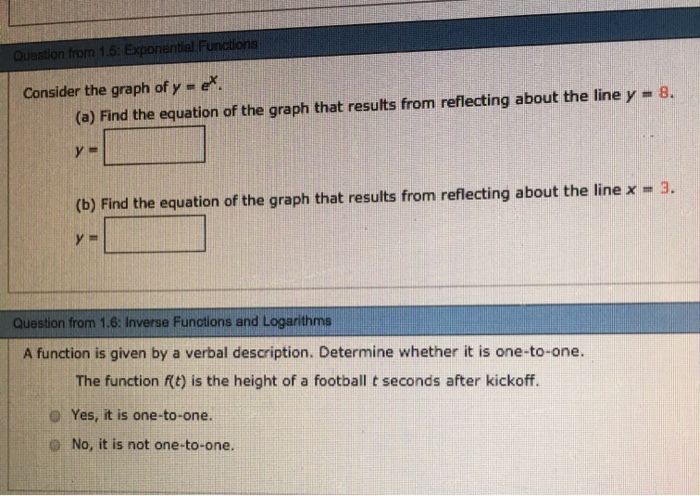Consider the graph of y m e. 8. (a) Find the equation of the graph that results from reflecting about the line y (b) Find the equation of the graph that results from reflecting about the line x -3. Question from 1.6: Inverse Functions and Logarithms A function is given by a verbal description. Determine whether it is one-to-one. The function f(t) is the height of a football t seconds after kickoff. D Yes, it is one-to-one. O No, it is not one-to-one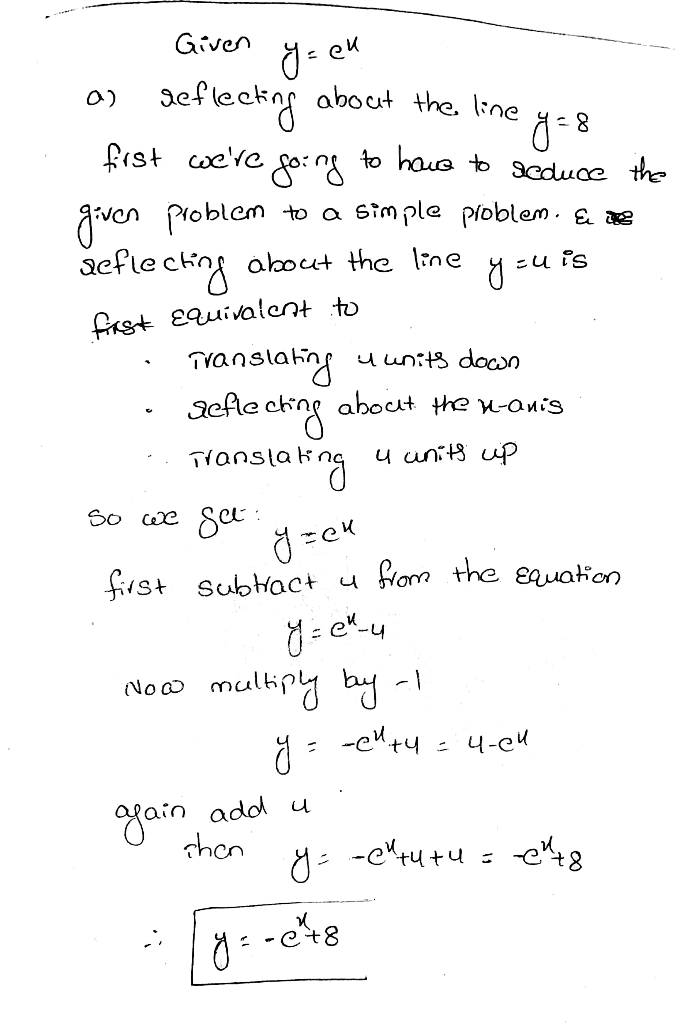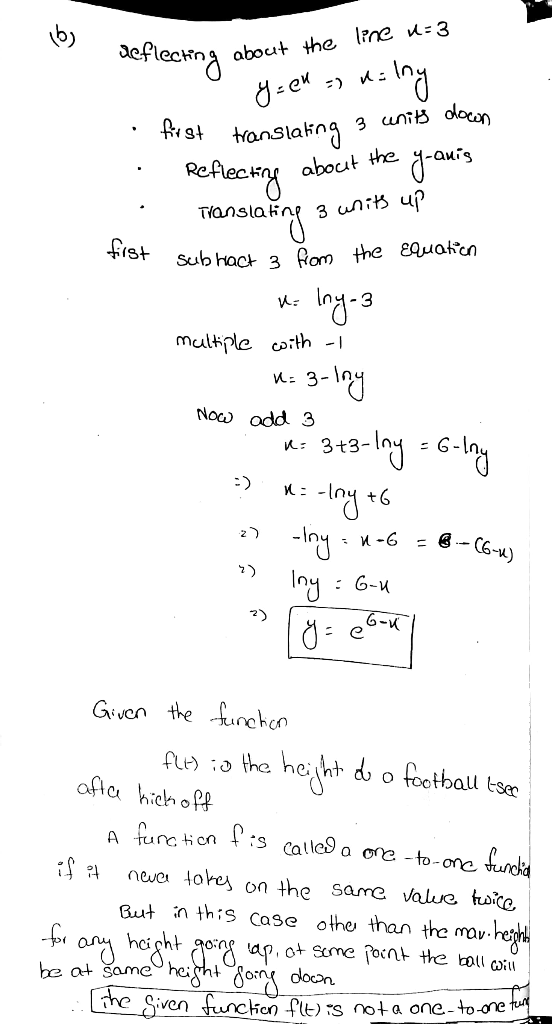##### Add Answer of: Consider the graph of y m e. 8. (a) Find the equation of the graph that results from reflecting about the line y (b...
Similar Homework Help Questions
• ### Starting with the graph of f(x) = 2^x, write the equation of the graph that results from reflecting f(x) about the y-axis

Starting with the graph of f(x) = 2^x, write the equation of the graph that results from reflecting f(x) about the y-axis. y = ?

• ### Equation of graph across line

Starting with the graph of y=ex , find the equation of tha graph that results froma) reflecting about the line y=4b) reflecting about the line x=2This is part of James Stewart Calculus Early Transcendentals 6E, chapter 1.5 problem 14 but it is explained incorrectly.

• ### (b) Consider the following function and graph. Find an equation of the tangent line to the graph...(b) Consider the following function and graph.Find an equation of the tangent line to the graph of the functionat point (0,1).y(x) =

• ### (a) Consider the following function and graph. Find an equation of the tangent line to the graph...(a) Consider the following function and graph.Find an equation of the tangent line to the graph of the functionat point (0,1).y(x) =Find an equation of the tangent line to the graph of the functionat point (0,1).y(x) =

• ### What is the equation for the line of symmetry for the graph of this function

What is the equation for the line of symmetry for the graph of this function? function y = x2 - 4x - 5,if you have a graphing calulator you can put it in there, or just graph it on a piece of paper using a T chart. find it by one of those.

• ### = y2(y? - 2), where y is a real dt dy Consider the differential equation 8.(10 points) function of t. (a) State the cri...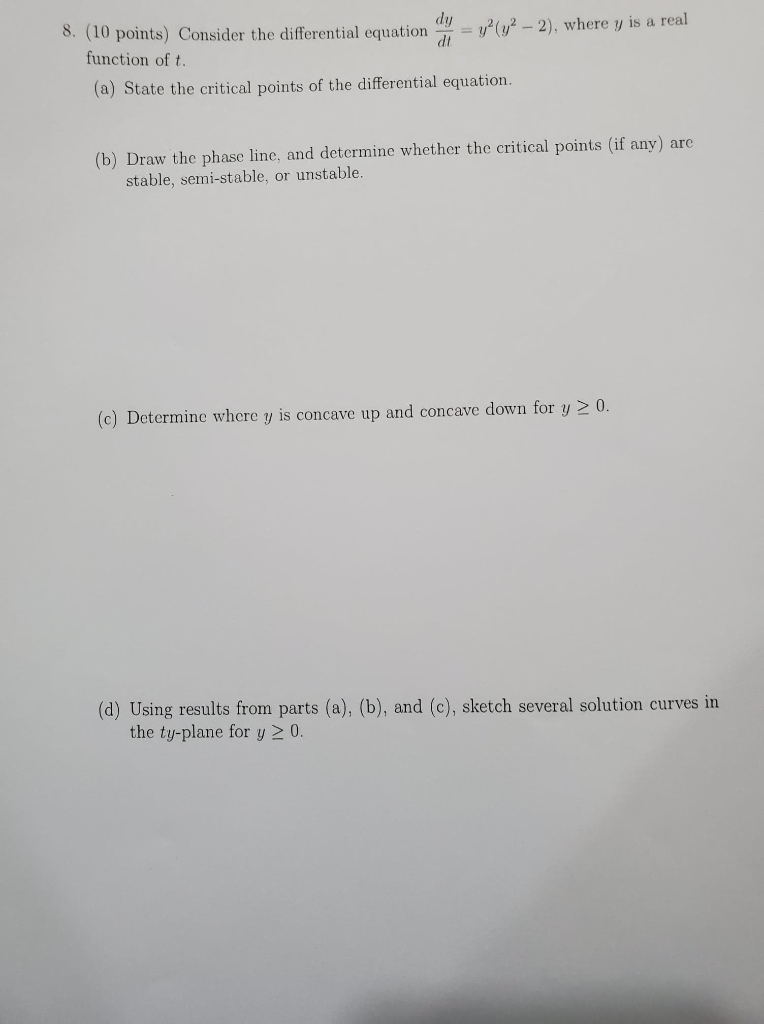= y2(y? - 2), where y is a real dt dy Consider the differential equation 8.(10 points) function of t. (a) State the critical points of the differential equation. (b) Draw the phase line, and determine whether the critical points (if any) are stable, semi-stable, or unstable. (c) Determine where y is concave up and concave down for y > 0. (d) Using results from parts (a), (b), and (c), sketch several solution curves in the ty-plane for y 2...

• ### 2. Use Definition to find the equation of the tangent line to the graph of the equation y- 1/2 at -2 3. Find the points on the graph of y2-/2 at which the tangent line is parallel to the line y -...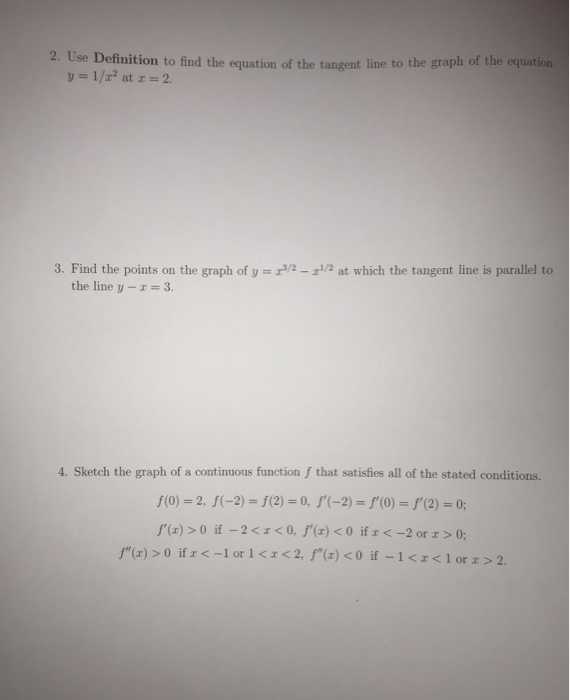2. Use Definition to find the equation of the tangent line to the graph of the equation y- 1/2 at -2 3. Find the points on the graph of y2-/2 at which the tangent line is parallel to the line y - 3. 4. Sketch the graph of a continuous function f that satisfies all of the stated conditions. f(0) 2, f(-2)- (2)-0, f(-2) f(O)-f'(2)-0 f"(z) > o if-2<zco, f,(z) < 0 if <-2 or x > 0; 2. Use...

Which three options are true about the quadratic equation?? y= 4t^2 + 16t + 18 A. The graph has a minimum. B. The graph of y crosses the horizontal axis at t = 10 and t = 20. C. The equation 4t^2 + 16 + 18 = 6 has one real solution. D. The function has a maximum at t = 10 E. The vertex is at (-2, 2) F. The function could represent the height y (in metres) of...

• ### For the graph of each function, find an equation of the line of symmetry and the coordinates of the vertex

For the graph of each function, find an equation of the line of symmetry and the coordinates of the vertex. Tell whether the value of the function at the vertex if a maximum or a minimum: a) y = -x^2 + 4x - 7; b) y = 2x^2 + 4x; c) y = -3x^2 - 18x + 5.

• ### consider the graph of y= f(x)

Let the function f(x) be given by f(x)=(35)/(x+4)and consider the graph of y = f(x).*What is the average rate of change from x_1 = 2 to x_2 = 5?*What are the coordinates of the point that is on the graph when x=2?*What are the coordinates of the point that is on the graph when x=5?*The name of the line which connects the two points you just found is called the secant line. Find the equation of the secant line in...

Need Online Homework Help?